### 15.2.1.1 The discrete case

Before entering the continuous realm, the concepts will first be described for discrete planning, which is often easier to understand. Recall from Section 2.3 that if,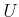, and the stages are discrete, then optimal planning can be performed by using value iteration or Dijkstra's algorithm on the search graph. The stationary, optimal cost-to-go function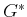can be used as a navigation function that encodes the optimal feedback plan. This was suggested in Section 8.2.2, and an example was shown in Figure 8.3.

Suppose thathas been computed under Formulation 8.1 (or Formulation 2.3). Let the state transition equation be denoted as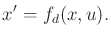(15.5)

The dynamic programming recurrence foris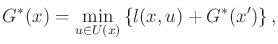(15.6)

which may already be considered as a discrete form of the Hamilton-Jacobi-Bellman equation. To gain some insights into the coming concepts, however, some further manipulations will be performed.

Let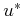denote the optimal action that is applied in the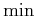of (15.6). Imagine thatis hypothesized as the optimal action but needs to be tested in (15.6) to make sure. If it is truly optimal, then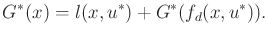(15.7)

This can already be considered as a discrete form of the Pontryagin minimum principle, which will appear in Section 15.2.3. By rearranging terms, a nice interpretation is obtained: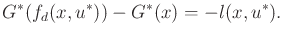(15.8)

In a single stage, the optimal cost-to-go drops by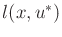whenis used as a navigation function (multiply (15.8) by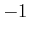). The optimal single-stage cost is revealed precisely when taking one step toward the goal along the optimal path. This incremental change in the cost-to-go function while moving in the best direction forms the basis of both the HJB equation and the minimum principle.

Steven M LaValle 2020-08-14